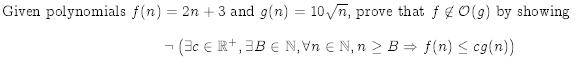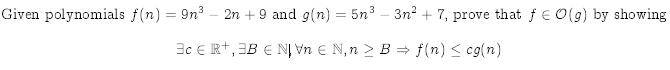# Need help disproving this

Melodia

## Homework Statement

Thanks for the help on the last problem. Here is the final problem set I'm stuck on:## The Attempt at a Solution

To me it seems that there will always be a positive c so that cg(n) is greater or equal to f(n). No matter how large n is, since there's no limit to how large c can be (can even be a decimal), wouldn't that always be possible?

Thanks.

Mentor
First off, g is not a polynomial, as it is not the sum of multiples of integral powers of n. It might be helpful for you to graph the two functions, because you would see that for n larger than about 20, the linear function dominates the other function. Although f(n) = 2n + 3 is a linear function and grows at only a constant rate, that rate is larger than that of the other function, for large enough n.

Melodia
Oooh I see. Since c is fixed and chosen before the n, then there will always be an n that contradicts the statement right?

I'm guessing the same thing applies to this other problem right?Last edited:
Staff Emeritus